# fitcsvm

## 语法

Mdl = fitcsvm(Tbl,ResponseVarName)
Mdl = fitcsvm(Tbl,formula)
Mdl = fitcsvm(Tbl,Y)
Mdl = fitcsvm(X,Y)
Mdl = fitcsvm(___,Name,Value)

## 说明

fitcsvm 基于低维或中维预测变量数据集训练或交叉验证一类和二类（二元）分类的支持向量机 (SVM) 模型。fitcsvm 支持使用核函数映射预测变量数据，并支持序列最小优化 (SMO)、迭代单点数据算法 (ISDA) 或 L1 软边距最小化（二次规划目标函数最小化）。

Mdl = fitcsvm(Tbl,ResponseVarName) 返回训练的支持向量机 (SVM) 分类器 Mdl。该分类器使用表 Tbl 中包含的样本数据进行训练。ResponseVarName 是 Tbl 中变量的名称，该变量包含一类或二类分类的类标签。如果类标签变量只包含一个类（例如，由 1 组成的向量），fitcsvm 会训练一类分类模型。否则，该函数将训练二类分类模型。
Mdl = fitcsvm(Tbl,formula) 返回训练的 SVM 分类器。该分类器使用表 Tbl 中包含的样本数据进行训练。formula 是用于拟合 Mdl 的解释模型，该模型由 Tbl 中的响应和一部分预测变量构成。
Mdl = fitcsvm(Tbl,Y) 返回训练的 SVM 分类器。该分类器使用表 Tbl 中的预测变量和向量 Y 中的类标签进行训练。

Mdl = fitcsvm(X,Y) 返回训练的 SVM 分类器，该分类器使用矩阵 X 中的预测变量和向量 Y 中的一类或二类分类的类标签进行训练。

Mdl = fitcsvm(___,Name,Value) 可在前面语法中的输入参数外使用一个或多个名称-值对组参数指定选项。例如，您可以指定交叉验证的类型、误分类的代价以及分数变换函数的类型。

## 示例

load fisheriris inds = ~strcmp(species,'setosa'); X = meas(inds,3:4); y = species(inds);

SVMModel = fitcsvm(X,y)
SVMModel = ClassificationSVM ResponseName: 'Y' CategoricalPredictors: [] ClassNames: {'versicolor' 'virginica'} ScoreTransform: 'none' NumObservations: 100 Alpha: [24x1 double] Bias: -14.4149 KernelParameters: [1x1 struct] BoxConstraints: [100x1 double] ConvergenceInfo: [1x1 struct] IsSupportVector: [100x1 logical] Solver: 'SMO' Properties, Methods 

SVMModel 是经过训练的 ClassificationSVM 分类器。您可以查看 SVMModel 的属性。例如，使用圆点表示法查看类顺序。

classOrder = SVMModel.ClassNames
classOrder = 2x1 cell {'versicolor'} {'virginica' } 

sv = SVMModel.SupportVectors; figure gscatter(X(:,1),X(:,2),y) hold on plot(sv(:,1),sv(:,2),'ko','MarkerSize',10) legend('versicolor','virginica','Support Vector') hold off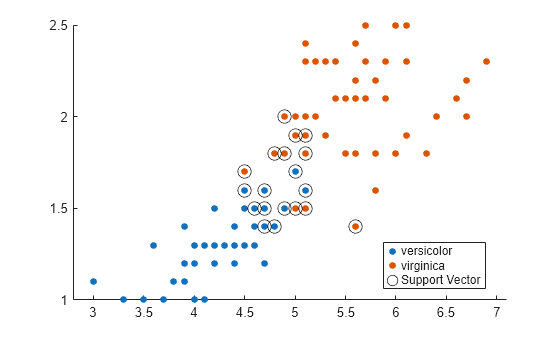load fisheriris; inds = ~strcmp(species,'versicolor'); X = meas(inds,1:2); s = species(inds);

SVMModel = fitcsvm(X,s);

SVMModel 是经过训练的 ClassificationSVM 分类器，其属性包括支持向量、线性预测变量系数和偏置项。

sv = SVMModel.SupportVectors; % Support vectors beta = SVMModel.Beta; % Linear predictor coefficients b = SVMModel.Bias; % Bias term

hold on gscatter(X(:,1),X(:,2),s) plot(sv(:,1),sv(:,2),'ko','MarkerSize',10)

SVMModel 分类器的最佳分离超平面是由 ${\beta }_{1}{X}_{1}+{\beta }_{2}{X}_{2}+b=0$ 指定的直线。将两个物种之间的决策边界绘制为一条实线。

X1 = linspace(min(X(:,1)),max(X(:,1)),100); X2 = -(beta(1)/beta(2)*X1)-b/beta(2); plot(X1,X2,'-')

m = 1/sqrt(beta(1)^2 + beta(2)^2); % Margin half-width X1margin_low = X1+beta(1)*m^2; X2margin_low = X2+beta(2)*m^2; X1margin_high = X1-beta(1)*m^2; X2margin_high = X2-beta(2)*m^2; plot(X1margin_high,X2margin_high,'b--') plot(X1margin_low,X2margin_low,'r--') xlabel('X_1 (Sepal Length in cm)') ylabel('X_2 (Sepal Width in cm)') legend('setosa','virginica','Support Vector', ... 'Boundary Line','Upper Margin','Lower Margin') hold off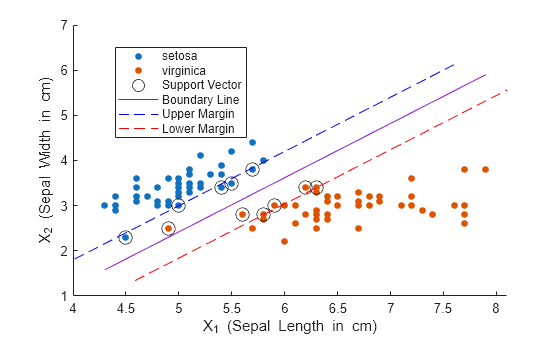load ionosphere rng(1); % For reproducibility

SVMModel = fitcsvm(X,Y,'Standardize',true,'KernelFunction','RBF',... 'KernelScale','auto');

SVMModel 是经过训练的 ClassificationSVM 分类器。

CVSVMModel = crossval(SVMModel);

CVSVMModelClassificationPartitionedModel 交叉验证的分类器。

classLoss = kfoldLoss(CVSVMModel)
classLoss = 0.0484 

load fisheriris X = meas(:,1:2); y = ones(size(X,1),1);

rng(1); SVMModel = fitcsvm(X,y,'KernelScale','auto','Standardize',true,... 'OutlierFraction',0.05);

SVMModel 是经过训练的 ClassificationSVM 分类器。默认情况下，软件使用高斯核进行一类学习。

svInd = SVMModel.IsSupportVector; h = 0.02; % Mesh grid step size [X1,X2] = meshgrid(min(X(:,1)):h:max(X(:,1)),... min(X(:,2)):h:max(X(:,2))); [~,score] = predict(SVMModel,[X1(:),X2(:)]); scoreGrid = reshape(score,size(X1,1),size(X2,2)); figure plot(X(:,1),X(:,2),'k.') hold on plot(X(svInd,1),X(svInd,2),'ro','MarkerSize',10) contour(X1,X2,scoreGrid) colorbar; title('{\bf Iris Outlier Detection via One-Class SVM}') xlabel('Sepal Length (cm)') ylabel('Sepal Width (cm)') legend('Observation','Support Vector') hold off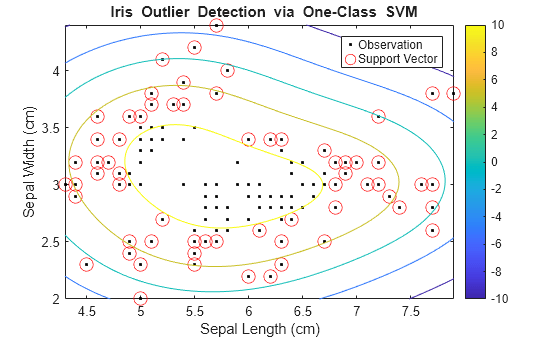CVSVMModel = crossval(SVMModel); [~,scorePred] = kfoldPredict(CVSVMModel); outlierRate = mean(scorePred<0)
outlierRate = 0.0467 

load fisheriris X = meas(:,3:4); Y = species;

figure gscatter(X(:,1),X(:,2),Y); h = gca; lims = [h.XLim h.YLim]; % Extract the x and y axis limits title('{\bf Scatter Diagram of Iris Measurements}'); xlabel('Petal Length (cm)'); ylabel('Petal Width (cm)'); legend('Location','Northwest');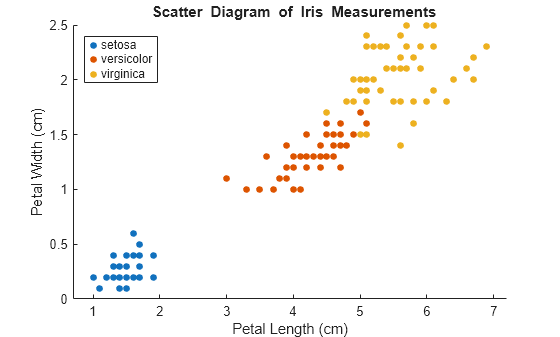1. 创建一个逻辑向量 (indx)，指示观测值是否为该类的成员。

2. 使用预测变量数据和 indx 训练 SVM 分类器。

3. 将该分类器存储在元胞数组的一个元胞中。

SVMModels = cell(3,1); classes = unique(Y); rng(1); % For reproducibility for j = 1:numel(classes) indx = strcmp(Y,classes(j)); % Create binary classes for each classifier SVMModels{j} = fitcsvm(X,indx,'ClassNames',[false true],'Standardize',true,... 'KernelFunction','rbf','BoxConstraint',1); end

SVMModels 是 3×1 元胞数组，其中每个元胞包含一个 ClassificationSVM 分类器。对于每个元胞，正类分别是 setosa、versicolor 和 virginica。

d = 0.02; [x1Grid,x2Grid] = meshgrid(min(X(:,1)):d:max(X(:,1)),... min(X(:,2)):d:max(X(:,2))); xGrid = [x1Grid(:),x2Grid(:)]; N = size(xGrid,1); Scores = zeros(N,numel(classes)); for j = 1:numel(classes) [~,score] = predict(SVMModels{j},xGrid); Scores(:,j) = score(:,2); % Second column contains positive-class scores end

Scores 的每行包含三个分数。分数最高的元素的索引是新类观测值最可能属于的类的索引。

[~,maxScore] = max(Scores,[],2);

figure h(1:3) = gscatter(xGrid(:,1),xGrid(:,2),maxScore,... [0.1 0.5 0.5; 0.5 0.1 0.5; 0.5 0.5 0.1]); hold on h(4:6) = gscatter(X(:,1),X(:,2),Y); title('{\bf Iris Classification Regions}'); xlabel('Petal Length (cm)'); ylabel('Petal Width (cm)'); legend(h,{'setosa region','versicolor region','virginica region',... 'observed setosa','observed versicolor','observed virginica'},... 'Location','Northwest'); axis tight hold off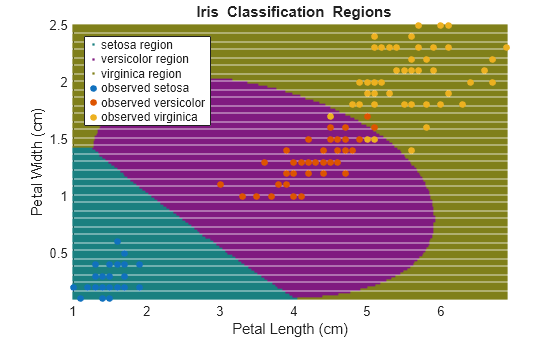load ionosphere

rng default Mdl = fitcsvm(X,Y,'OptimizeHyperparameters','auto', ... 'HyperparameterOptimizationOptions',struct('AcquisitionFunctionName', ... 'expected-improvement-plus'))
|=====================================================================================================| | Iter | Eval | Objective | Objective | BestSoFar | BestSoFar | BoxConstraint| KernelScale | | | result | | runtime | (observed) | (estim.) | | | |=====================================================================================================| | 1 | Best | 0.20513 | 16.349 | 0.20513 | 0.20513 | 64.836 | 0.0015729 | | 2 | Accept | 0.35897 | 0.14027 | 0.20513 | 0.21471 | 0.036335 | 5.5755 | | 3 | Best | 0.13105 | 6.6774 | 0.13105 | 0.14133 | 0.0022147 | 0.0023957 | | 4 | Accept | 0.35897 | 0.19112 | 0.13105 | 0.13109 | 5.1259 | 98.62 | | 5 | Accept | 0.1339 | 14.128 | 0.13105 | 0.13111 | 0.0011147 | 0.0010089 | | 6 | Accept | 0.13675 | 2.8413 | 0.13105 | 0.13117 | 0.0010151 | 0.0059137 | | 7 | Best | 0.12821 | 8.0524 | 0.12821 | 0.12827 | 0.0010563 | 0.0022307 | | 8 | Accept | 0.1339 | 7.5899 | 0.12821 | 0.13053 | 0.0010113 | 0.0026572 | | 9 | Accept | 0.12821 | 7.7937 | 0.12821 | 0.12991 | 0.0010145 | 0.0018037 | | 10 | Accept | 0.12821 | 8.4811 | 0.12821 | 0.1294 | 0.0010049 | 0.0018883 | | 11 | Accept | 0.13675 | 15.109 | 0.12821 | 0.12842 | 994.04 | 0.19205 | | 12 | Accept | 0.1339 | 12.793 | 0.12821 | 0.1288 | 8.1763 | 0.047242 | | 13 | Accept | 0.22507 | 16.693 | 0.12821 | 0.12804 | 983.48 | 0.067705 | | 14 | Accept | 0.13105 | 0.39394 | 0.12821 | 0.12807 | 0.058846 | 0.073767 | | 15 | Accept | 0.12821 | 4.4698 | 0.12821 | 0.12806 | 22.877 | 0.2836 | | 16 | Accept | 0.12821 | 5.4563 | 0.12821 | 0.12811 | 0.12835 | 0.020261 | | 17 | Accept | 0.13675 | 12.678 | 0.12821 | 0.12789 | 959.28 | 0.57912 | | 18 | Accept | 0.12821 | 2.72 | 0.12821 | 0.12809 | 1.6281 | 0.087712 | | 19 | Accept | 0.12821 | 0.15632 | 0.12821 | 0.1281 | 0.0010071 | 0.029923 | | 20 | Accept | 0.12821 | 2.5345 | 0.12821 | 0.12794 | 0.37931 | 0.04231 | |=====================================================================================================| | Iter | Eval | Objective | Objective | BestSoFar | BestSoFar | BoxConstraint| KernelScale | | | result | | runtime | (observed) | (estim.) | | | |=====================================================================================================| | 21 | Accept | 0.13675 | 12.566 | 0.12821 | 0.12792 | 218.45 | 0.28692 | | 22 | Accept | 0.1396 | 0.46859 | 0.12821 | 0.12816 | 0.0084263 | 0.024097 | | 23 | Accept | 0.1339 | 0.17073 | 0.12821 | 0.12795 | 0.0011093 | 0.11727 | | 24 | Accept | 0.12821 | 14.216 | 0.12821 | 0.12798 | 0.76711 | 0.0086394 | | 25 | Accept | 0.13675 | 0.36176 | 0.12821 | 0.12805 | 0.83585 | 0.25134 | | 26 | Accept | 0.17949 | 16.549 | 0.12821 | 0.12796 | 0.11898 | 0.0010906 | | 27 | Accept | 0.1339 | 13.043 | 0.12821 | 0.12797 | 1.3574 | 0.020097 | | 28 | Accept | 0.13675 | 11.003 | 0.12821 | 0.12803 | 0.088329 | 0.0068614 | | 29 | Accept | 0.12821 | 4.7252 | 0.12821 | 0.1286 | 7.8795 | 0.16358 | | 30 | Best | 0.11966 | 0.20019 | 0.11966 | 0.11999 | 0.0010805 | 0.060691 |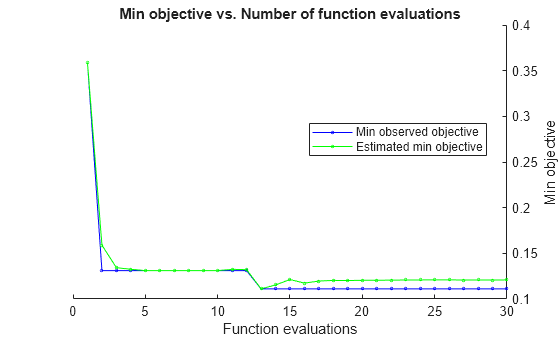__________________________________________________________ Optimization completed. MaxObjectiveEvaluations of 30 reached. Total function evaluations: 30 Total elapsed time: 246.8684 seconds Total objective function evaluation time: 218.5525 Best observed feasible point: BoxConstraint KernelScale _____________ ___________ 0.0010805 0.060691 Observed objective function value = 0.11966 Estimated objective function value = 0.11999 Function evaluation time = 0.20019 Best estimated feasible point (according to models): BoxConstraint KernelScale _____________ ___________ 0.0010805 0.060691 Estimated objective function value = 0.11999 Estimated function evaluation time = 0.16467 
Mdl = ClassificationSVM ResponseName: 'Y' CategoricalPredictors: [] ClassNames: {'b' 'g'} ScoreTransform: 'none' NumObservations: 351 HyperparameterOptimizationResults: [1x1 BayesianOptimization] Alpha: [118x1 double] Bias: -2.5864 KernelParameters: [1x1 struct] BoxConstraints: [351x1 double] ConvergenceInfo: [1x1 struct] IsSupportVector: [351x1 logical] Solver: 'SMO' Properties, Methods 

## 输入参数

（可选）Tbl 可以包含一个对应于响应变量的列和一个对应于观测值权重的列。

• 响应变量必须为分类数组、字符数组或字符串数组、逻辑向量或数值向量，或者字符向量元胞数组。

• fitcsvm 仅支持一类和二类（二元）分类。或者响应变量最多只能包含两个不同类，或您必须使用 ClassNames 名称-值参数指定一个或两个用于训练的类。有关多类学习的信息，请参阅 fitcecoc

• 使用 ClassNames 名称-值参数在响应变量中指定类的顺序是很好的做法。

• 权重列必须为数值向量。

• 您必须使用 ResponseVarNameformulaTbl 中指定响应变量，并使用 WeightsTbl 中指定观测值权重。

• 通过使用 ResponseVarName 指定响应变量 - fitcsvm 将其余变量用作预测变量。要使用 Tbl 中其余变量的子集作为预测变量，请使用 PredictorNames 指定预测变量。

• 使用 formula 定义模型设定 - fitcsvm 使用 Tbl 中变量的子集作为预测变量和响应变量，如 formula 中所指定的那样。

• fitcsvm 仅支持一类和二类（二元）分类。或者 Y 最多只能包含两个不同类，或您必须使用 ClassNames 名称-值参数指定一个或两个用于训练的类。有关多类学习的信息，请参阅 fitcecoc

• Y 的长度和 TblX 的行数必须相等。

• 如果 Y 是字符数组，则每个标签必须对应于数组的一行。

• 使用 ClassNames 名称-值对组参数来指定类顺序是很好的做法。

X 的每行对应一个观测值（也称为实例或示例），每列对应一个预测变量（也称为特征）。

Y 的长度和 X 的行数必须相等。

### 名称-值参数

SVM 选项

'gaussian''rbf'高斯或径向基函数 (RBF) 核，默认用于一类学习

$G\left({x}_{j},{x}_{k}\right)=\mathrm{exp}\left(-{‖{x}_{j}-{x}_{k}‖}^{2}\right)$

'linear'线性核，默认用于二类学习

$G\left({x}_{j},{x}_{k}\right)={x}_{j}\prime {x}_{k}$

'polynomial'多项式核。使用 'PolynomialOrder',q 指定 q 次多项式核。

$G\left({x}_{j},{x}_{k}\right)={\left(1+{x}_{j}\prime {x}_{k}\right)}^{q}$

function G = kernel(U,V)

• U 是 m×p 矩阵。列对应于预测变量，行对应于观测值。

• V 是 n×p 矩阵。列对应于预测变量，行对应于观测值。

• GUV 行的 m×n Gram 矩阵

kernel.m 必须在 MATLAB 路径上。

• 如果您指定 'auto'，则软件使用启发式过程选择适当的尺度因子。这种启发式过程使用二次抽样，因此不同调用的估计值可能不同。因此，为了重现结果，请在训练前使用 rng 设置随机数种子。

• 如果您指定 KernelScale 和您自己的核函数，例如 'KernelFunction','kernel'，则软件会引发错误。您必须在 kernel 中应用尺度缩放。

• 0，如果求解器是 SMO（也就是说，您设置 'Solver','SMO'

• 0.1，如果求解器是 ISDA（也就是说，您设置 'Solver','ISDA'

• 软件按对应的加权列均值和标准差对每个预测变量（XTbl）进行中心化并缩放。有关加权标准化的详细信息，请参阅算法。MATLAB 不对为分类预测变量生成的虚拟变量列中包含的数据进行标准化。

• 软件使用经过标准化的预测变量训练分类器，但会将未标准化的预测变量以矩阵或表形式存储在分类器属性 X 中。

'ISDA'迭代单数据算法（请参阅 
'L1QP'使用 quadprog (Optimization Toolbox) 通过二次规划实现 L1 软边距最小化。此选项需要 Optimization Toolbox™ 许可证。有关详细信息，请参阅二次规划定义 (Optimization Toolbox)
'SMO'序列最小优化（请参阅 

alpha 系数的初始估计值，指定为以逗号分隔的对组，其中包含 'Alpha' 和非负值的数值向量。Alpha 的长度必须等于 X 的行数。

• 'Alpha' 的每个元素对应于 X 中的一个观测值。

• 'Alpha' 不能包含任何 NaN

• 如果您指定 'Alpha' 和任一交叉验证名称-值对组参数（'CrossVal''CVPartition''Holdout''KFold''Leaveout'），软件将返回错误。

idx = ~isundefined(categorical(Y)); Y = Y(idx,:); X = X(idx,:); alpha = alpha(idx);

• 0.5*ones(size(X,1),1)（用于一类学习）

• zeros(size(X,1),1)（用于二类学习）

true在每次迭代中，如果 αj 接近 0 或接近 Cj，则 MATLAB 分别将 αj 设置为 0 或 Cj
falseMATLAB 在优化过程中不更改 alpha 系数。

MATLAB 将 α 的最终值存储在经过训练的 SVM 模型对象的 Alpha 属性中。

ClipAlphas 会影响 SMO 和 ISDA 收敛。

Nu 用于在确保大多数训练样本为正类和尽量减小分数函数权重之间进行权衡。

• 对于二类学习，软件实现稳健学习。换句话说，当优化算法收敛时，软件尝试删除 100*outlierfraction% 的观测值。删除的观测值对应于幅值较大的梯度。

• 对于一类学习，软件会使用合适的偏差项，使得训练集中 outlierfraction 的观测值具有负分数。

0软件不显示或保存收敛信息。
1软件显示诊断消息，并每隔 numprint 次迭代保存收敛条件，其中 numprint 是名称-值对组参数 'NumPrint' 的值。
2软件显示诊断消息，并在每次迭代时保存收敛条件。

true 条目表示对应的预测变量是分类变量。向量的长度为 p

"all"所有预测变量均为分类预测变量。

• 指定训练期间类的顺序。

• 指定对应于类顺序的任何输入或输出参数维度的顺序。例如，使用 ClassNames 指定 Cost 的维度的顺序或 predict 返回的分类分数的列顺序。

• 选择用于训练的类的子集。例如，假设 Y 中所有不同类名的集合是 ["a","b","c"]。要仅使用来自 "a""c" 类的观测值训练模型，请指定 "ClassNames",["a","c"]

ClassNames 的默认值为 TblY 中的响应变量的所有不同类名的集合。

• 如果您指定方阵 Cost 且观测值的真实类是 i，则 Cost(i,j) 是将一个点分类到 j 类的代价。也就是说，行对应于真实类，列对应于预测的类。要为 Cost 的对应行和列指定类顺序，则还需指定 ClassNames 名称-值对组参数。

• 如果您指定结构体 S，则它必须有两个字段：

• S.ClassNames，其中包含类名作为一个变量，变量的数据类型与 Y 相同。

• S.ClassificationCosts，其中包含按 S.ClassNames 中的顺序排列行和列的代价矩阵

• 如果您提供 XY，则可以使用 PredictorNamesX 中的预测变量指定名称。

• PredictorNames 中名称的顺序必须对应于 X 中列的顺序。也就是说，PredictorNames{1}X(:,1) 的名称，PredictorNames{2}X(:,2) 的名称，依此类推。此外，size(X,2)numel(PredictorNames) 必须相等。

• 默认情况下，PredictorNames{'x1','x2',...}

• 如果您提供 Tbl，则您可以使用 PredictorNames 来选择在训练中使用哪些预测变量。也就是说，fitcsvm 仅使用 PredictorNames 中的预测变量和训练过程中的响应变量。

• PredictorNames 必须为 Tbl.Properties.VariableNames 的子集，并且不能包含响应变量的名称。

• 默认情况下，PredictorNames 包含所有预测变量的名称。

• 最好仅使用 PredictorNamesformula（但不能同时使用两者）来指定训练的预测变量。

'empirical'类先验概率是 Y 中的类相对频数。
'uniform'所有类先验概率等于 1/K，其中 K 是类的数目。

• S.ClassNames 包含类名作为一个变量，变量的数据类型与 Y 相同。

• S.ClassProbs 包含对应先验概率的向量。软件会对向量的元素进行归一化，使其总和为 1

"doublelogit"1/(1 + e–2x)
"invlogit"log(x / (1 – x))
"ismax"将分数最高的类的分数设置为 1，并将所有其他类的分数设置为 0
"logit"1/(1 + e–x)
"none""identity"x（无变换）
"sign"–1 表示 x < 0
0 表示 x = 0
1 表示 x > 0
"symmetric"2x – 1
"symmetricismax"将分数最高的类的分数设置为 1，并将所有其他类的分数设置为 –1
"symmetriclogit"2/(1 + e–x) – 1

1. 随机选择并预留 p*100% 的数据作为验证数据，并使用其余数据训练模型。

2. 将经过训练的紧凑模型存储在交叉验证模型的 Trained 属性中。

1. 将数据随机分为 k 个数据集。

2. 对于每个数据集，预留用作验证数据的数据集，并使用其他 k – 1 数据集训练模型。

3. k 个紧凑的经过训练的模型存储在交叉验证模型的 Trained 属性中的 k×1 元胞向量中。

1. 预留作为验证数据的观测值，并使用其他 n - 1 个观测值训练模型。

2. 将 n 个紧凑的经过训练的模型存储在交叉验证模型的 Trained 属性中的 n×1 元胞向量中。

• 如果求解器是 SMO（例如，您设置 'Solver','SMO'），默认值为 1e-3

• 如果求解器是 ISDA（例如，您设置 'Solver','ISDA'），默认值 0

Karush-Kuhn-Tucker (KKT) 互补条件违规容限，指定为由 'KKTTolerance' 和非负标量组成的以逗号分隔的对组。

• 如果求解器是 SMO（例如，您设置 'Solver','SMO'），默认值为 0

• 如果求解器是 ISDA（例如，您设置 'Solver','ISDA'），默认值 1e-3

• 'none' - 不优化。

• 'auto' - 使用 {'BoxConstraint','KernelScale'}

• 'all' - 优化所有合格参数。

• 合格参数名称的字符串数组或元胞数组。

• optimizableVariable 对象的向量，通常是 hyperparameters 的输出。

'OptimizeHyperparameters' 的值会覆盖您使用其他名称-值参数指定的任何值。例如，将 'OptimizeHyperparameters' 设置为 'auto' 会导致 fitcsvm 优化与 'auto' 选项对应的超参数，并忽略超参数的任何指定值。

fitcsvm 的合格参数包括：

load fisheriris params = hyperparameters('fitcsvm',meas,species); params(1).Range = [1e-4,1e6];

params 作为 OptimizeHyperparameters 的值传递。

Optimizer
• 'bayesopt' - 使用贝叶斯优化。在内部，此设置调用 bayesopt

• 'gridsearch' - 使用网格搜索，每个维度有 NumGridDivisions 个值。

• 'randomsearch' - 在 MaxObjectiveEvaluations 个点中随机搜索。

'gridsearch' 基于网格的均匀无放回抽样以随机顺序进行搜索。优化后，您可以使用命令 sortrows(Mdl.HyperparameterOptimizationResults) 按网格顺序获得一个表。

'bayesopt'
AcquisitionFunctionName

• 'expected-improvement-per-second-plus'

• 'expected-improvement'

• 'expected-improvement-plus'

• 'expected-improvement-per-second'

• 'lower-confidence-bound'

• 'probability-of-improvement'

'expected-improvement-per-second-plus'
MaxObjectiveEvaluations目标函数计算的最大数量。对于 'bayesopt''randomsearch'30，对于 'gridsearch' 为整个网格
MaxTime

Inf
NumGridDivisions用于 'gridsearch'，表示每个维度中的值的数量。该值可以是由指定每个维度上值个数的正整数组成的向量，也可以是应用于所有维度的标量。对于分类变量，此字段被忽略。10
ShowPlots指示是否显示绘图的逻辑值。如果为 true，此字段绘制最佳观测目标函数值对迭代编号的图。如果使用贝叶斯优化（Optimizer'bayesopt'），则此字段还会绘制最佳估计目标函数值。最佳观测目标函数值和最佳估计目标函数值分别对应于迭代输出的 BestSoFar (observed)BestSoFar (estim.) 列中的值。您可以在 Mdl.HyperparameterOptimizationResults 的属性 ObjectiveMinimumTraceEstimatedObjectiveMinimumTrace 中找到这些值。如果问题包含一个或两个用于贝叶斯优化的优化参数，则 ShowPlots 还会绘制目标函数的模型对这些参数的图。true
SaveIntermediateResults指示当 Optimizer'bayesopt' 时是否保存结果的逻辑值。如果为 true，此字段将在每次迭代中覆盖名为 'BayesoptResults' 的工作区变量。变量是 BayesianOptimization 对象。false
Verbose

• 0 - 不显示迭代输出

• 1 - 显示迭代输出

• 2 - 显示带额外信息的迭代输出

1
UseParallel指示是否并行运行贝叶斯优化的逻辑值，这需要 Parallel Computing Toolbox™。由于并行时序的不可再现性，并行贝叶斯优化不一定产生可重现的结果。有关详细信息，请参阅 Parallel Bayesian Optimizationfalse
Repartition

false

CVPartitioncvpartition 对象，由 cvpartition 创建如果未指定交叉验证字段，则为 'Kfold',5
Holdout(0,1) 范围内的标量，表示保留比例。
Kfold大于 1 的整数。

## 限制

• fitcsvm 为一类或二类学习应用训练 SVM 分类器。要使用就有两个以上类的数据训练 SVM 分类器，请使用 fitcecoc

• fitcsvm 支持低维和中维数据集。对于高维数据集，请改用 fitclinear

## 详细信息

### Karush-Kuhn-Tucker (KKT) 互补条件

KKT 互补条件是最优非线性规划解所需的优化约束。

$\left\{\begin{array}{l}{\alpha }_{j}\left[{y}_{j}f\left({x}_{j}\right)-1+{\xi }_{j}\right]=0\\ {\xi }_{j}\left(C-{\alpha }_{j}\right)=0\end{array}$

，其中 $f\left({x}_{j}\right)=\varphi \left({x}_{j}\right)\prime \beta +b,$ ϕ 是核函数（请参阅 Gram 矩阵），ξj 是松弛变量。如果这些类完全可分离，则对于所有 j = 1,...,n，满足 ξj = 0。

### 一类学习

$0.5\sum _{jk}{\alpha }_{j}{\alpha }_{k}G\left({x}_{j},{x}_{k}\right)$

，且对于所有 j = 1、...、n，满足

$\sum {\alpha }_{j}=n\nu$

$0\le {\alpha }_{j}\le 1$。G(xj,xk) 的值在 Gram 矩阵的元素 (j,k) 中。

### 用于二类分类的支持向量机

SVM 二类分类算法搜索将数据分成两类的最优超平面。对于可分离类，最优超平面会最大化自身周围的边距（不包含任何观测值的空间），从而在正类和负类间创建边界。对于不可分离的类，目标是相同的，但是，如果有观测值位于其类边界的错误一侧，则算法会相应地对边距长度施加罚分。

$f\left(x\right)=x\prime \beta +b,$

• x 是观测值（对应于 X 的一行）。

• 向量 β 包含定义超平面的正交向量的系数（对应于 Mdl.Beta）。对于可分离的数据，最佳边距长度是 $2/‖\beta ‖.$

• b 是偏差项（对应于 Mdl.Bias）。

• 对于可分离的类，目标是最小化关于 β 和 b 的 $‖\beta ‖$，并且对于所有 j = 1、...、n，满足 yjf(xj) ≥ 1。这是针对可分离类的问题形式。

• 对于不可分离的类，算法会在遇到跨越类边界的观测值时使用松弛变量 (ξj) 对目标函数进行罚分。对于未跨越类边界的观测值，ξj = 0，否则 ξj ≥ 0。

目标是最小化关于 β、b 和 ξj$0.5{‖\beta ‖}^{2}+C\sum {\xi }_{j}$，对于所有 j = 1,..,n 和正标量框约束 C，满足 ${y}_{j}f\left({x}_{j}\right)\ge 1-{\xi }_{j}$${\xi }_{j}\ge 0$。这是针对不可分离类的原问题形式。

• 对于可分离的类，最小化关于 α1,...,αn

$0.5\sum _{j=1}^{n}\sum _{k=1}^{n}{\alpha }_{j}{\alpha }_{k}{y}_{j}{y}_{k}{x}_{j}\prime {x}_{k}-\sum _{j=1}^{n}{\alpha }_{j}$

，对于所有 j = 1,...,n，满足 $\sum {\alpha }_{j}{y}_{j}=0$，αj ≥ 0，且满足 Karush-Kuhn-Tucker (KKT) 互补条件

• 对于不可分离的类，目标与可分离的类相同，不同之处是所有 j = 1、...、n 需满足附加条件 $0\le {\alpha }_{j}\le C$

$\stackrel{^}{f}\left(x\right)=\sum _{j=1}^{n}{\stackrel{^}{\alpha }}_{j}{y}_{j}x\prime {x}_{j}+\stackrel{^}{b}.$

$\stackrel{^}{b}$ 是偏差的估计值，${\stackrel{^}{\alpha }}_{j}$ 是向量 $\stackrel{^}{\alpha }$ 的第 j 个估计值，j = 1,...,n。写为这种形式时，score 函数不再需要原问题形式中的 β 估计值。

SVM 算法使用 $\text{sign}\left(\stackrel{^}{f}\left(z\right)\right).$ 对新观测值 z 进行分类

$0.5\sum _{j=1}^{n}\sum _{k=1}^{n}{\alpha }_{j}{\alpha }_{k}{y}_{j}{y}_{k}G\left({x}_{j},{x}_{k}\right)-\sum _{j=1}^{n}{\alpha }_{j}$

$\stackrel{^}{f}\left(x\right)=\sum _{j=1}^{n}{\stackrel{^}{\alpha }}_{j}{y}_{j}G\left(x,{x}_{j}\right)+\stackrel{^}{b}.$

## 提示

• 除非您的数据集很大，否则请始终尝试标准化预测变量（请参阅 Standardize）。标准化可使预测变量不受其测量尺度的影响。

• 使用 KFold 名称-值对组参数进行交叉验证是很好的做法。交叉验证结果决定 SVM 分类器的泛化能力。

• 对于一类学习：

• 名称-值对组参数 Alpha 的默认设置可能导致较长的训练时间。要加快训练速度，可将 Alpha 设置为主要由 0 组成的向量。

• 将名称-值对组参数 Nu 设置为更接近 0 的值可产生更少的支持向量，从而获得更平滑但粗糙的决策边界。

• 支持向量中的稀疏性是 SVM 分类器的一个理想属性。要减少支持向量的数量，可将 BoxConstraint 设置为较大的值。此操作会增加训练时间。

• 为了获得最佳训练时间，请将 CacheSize 设置为计算机允许的最大内存限制。

• 如果您期望支持向量比训练集中的观测值少得多，则您可以使用名称-值对组参数 'ShrinkagePeriod' 缩小活动集，从而显著加快收敛速度。指定 'ShrinkagePeriod',1000 是很好的做法。

• 远离决策边界的重复观测值不影响收敛。然而，决策边界附近出现的少数几个重复的观测值可能会大幅减慢收敛速度。在以下情况下，要加快收敛速度，请指定 'RemoveDuplicates',true

• 您的数据集包含许多重复的观测值。

• 您怀疑有几个重复的观测值接近决策边界。

要在训练期间保留原始数据集，fitcsvm 必须临时分别存储两个数据集：原始数据集和没有重复观测值的数据集。因此，如果您为包含少量重复观测值的数据集指定 true，则 fitcsvm 消耗的内存将接近原始数据的两倍。

• 在训练模型后，您可以生成预测新数据标签的 C/C++ 代码。生成 C/C++ 代码需要 MATLAB Coder™。有关详细信息，请参阅Introduction to Code Generation

## 算法

• 有关 SVM 二类分类算法的数学公式，请参阅用于二类分类的支持向量机了解支持向量机

• NaN<undefined>、空字符向量 ('')、空字符串 ("") 和 <missing> 值表示缺失值。fitcsvm 会删除对应于缺失响应的整行数据。在计算总权重时（请参阅下几项内容），如果某权重所对应的观测值至少具有一个缺失变量，则 fitcsvm 忽略该权重。此操作会导致平衡类问题中出现不平衡的先验概率。因此，观测值框约束可能不等于 BoxConstraint

• 如果您指定 CostPriorWeights 名称-值参数，则输出模型对象会将指定的值分别存储在 CostPriorW 属性中。Cost 属性存储用户指定的代价矩阵 (C)，无需进行任何修改。PriorW 属性分别存储归一化后的先验概率和观测值权重。对于模型训练，软件会更新先验概率和观测值权重，以纳入代价矩阵中所述的罚分。有关详细信息，请参阅Misclassification Cost Matrix, Prior Probabilities, and Observation Weights

请注意，CostPrior 名称-值参数用于二类学习。对于一类学习，CostPrior 属性分别存储 01

• 对于二类学习，fitcsvm 为训练数据中的每个观测值指定一个框约束。观察值 j 的框约束的公式是

${C}_{j}=n{C}_{0}{w}_{j}^{\ast },$

，其中 C0 是初始框约束（请参阅 BoxConstraint 名称-值参数），而 wj* 是观测值 j 的由 CostPrior 调整的观测值权重。有关观测值权重的详细信息，请参阅Adjust Prior Probabilities and Observation Weights for Misclassification Cost Matrix

• 如果您将 Standardize 指定为 true 并设置 CostPriorWeights 名称-值参数，则 fitcsvm 会使用对应于预测变量的加权均值和加权标准差对预测变量进行标准化。也就是说，fitcsvm 使用

${x}_{j}^{\ast }=\frac{{x}_{j}-{\mu }_{j}^{\ast }}{{\sigma }_{j}^{\ast }},$

标准化预测变量 j（xj，其中 xjk 是预测变量 j（列）的观测值 k（行），且

$\begin{array}{c}{\mu }_{j}^{\ast }=\frac{1}{\sum _{k}{w}_{k}^{*}}\sum _{k}{w}_{k}^{*}{x}_{jk},\\ {\left({\sigma }_{j}^{\ast }\right)}^{2}=\frac{{v}_{1}}{{v}_{1}^{2}-{v}_{2}}\sum _{k}{w}_{k}^{*}{\left({x}_{jk}-{\mu }_{j}^{\ast }\right)}^{2},\\ {v}_{1}=\sum _{j}{w}_{j}^{*},\\ {v}_{2}=\sum _{j}{\left({w}_{j}^{*}\right)}^{2}.\end{array}$

• 假设 p 是您预期在训练数据中的离群值比例，并且您设置了 'OutlierFraction',p

• 对于一类学习，软件会训练偏差项，使得训练数据中 100p% 的观测值具有负分数。

• 对于二类学习，软件会实施稳健学习。换句话说，当优化算法收敛时，软件会尝试删除 100p% 的观测值。删除的观测值对应于幅值较大的梯度。

• 如果预测变量数据包含分类变量，则软件通常会对这些变量进行完全虚拟变量编码。软件为每个分类变量的每个水平创建一个虚拟变量。

• PredictorNames 属性为每个原始预测变量名称存储一个元素。例如，假设有三个预测变量，其中一个是具有三个水平的分类变量。那么 PredictorNames 是 1×3 个字符向量元胞数组，其中包含预测变量的原始名称。

• ExpandedPredictorNames 属性为每个预测变量（包括虚拟变量）存储一个元素。例如，假设有三个预测变量，其中一个是具有三个水平的分类变量。那么 ExpandedPredictorNames 就是 1×5 字符向量数组，其中包含预测变量和新虚拟变量的名称。

• 同样，Beta 属性为每个预测变量（包括虚拟变量）存储一个 beta 系数。

• SupportVectors 属性存储支持向量的预测变量值，包括虚拟变量。例如，假设有 m 个支持向量和三个预测变量，其中一个预测变量是具有三个水平的分类变量。那么 SupportVectors 就是 n×5 矩阵。

• X 属性将训练数据存储为原始输入，不包括虚拟变量。当输入为表时，X 仅包含用作预测变量的列。

• 对于表中指定的预测变量，如果任何变量包含经过排序的（有序）类别，软件会对这些变量使用有序编码。

• 对于具有 k 个有序水平的变量，软件会创建 k – 1 个虚拟变量。在第 j 个虚拟变量中，前 j 个水平的对应值为 –1；从 j + 1 至 k 的水平的对应值为 +1

• 存储在 ExpandedPredictorNames 属性中的虚拟变量的名称使用值 +1 指示第一个水平。软件会为虚拟变量另外存储 k – 1 个预测变量名称，包括水平 2、3、...、k 的名称。

• 所有求解器都实现 L1 软边距最小化算法。

• 对于一类学习，软件估计 Lagrange 乘数 α1、...、αn，满足

$\sum _{j=1}^{n}{\alpha }_{j}=n\nu .$

## 替代功能

• fitcsvm 函数相比，ocsvm 函数针对异常检测提供的首选工作流更简单。

• ocsvm 函数返回 OneClassSVM 对象、异常指标和异常分数。您可以使用输出来识别训练数据中的异常。要找出新数据中的异常，可以使用 OneClassSVMisanomaly 对象函数。isanomaly 函数返回新数据的异常指标和分数。

• fitcsvm 函数支持一类和二类分类。如果类标签变量只包含一个类（例如，由 1 组成的向量），fitcsvm 会训练一类分类模型并返回 ClassificationSVM 对象。要识别异常，您必须首先使用 ClassificationSVMresubPredictpredict 对象函数计算异常分数，然后通过找出具有负分数的观测值来识别异常。

• 请注意，大的正异常分数表示 ocsvm 中存在异常，而负分数表示 ClassificationSVMpredict 中存在异常。

• ocsvm 函数基于 SVM 的原问题形式找到决策边界，而 fitcsvm 函数基于 SVM 的对偶问题形式找到决策边界。

• 对于大型数据集（大型 n），ocsvm 中求解器的计算开销低于 fitcsvm 中的求解器。与 fitcsvm 中需要计算 n×n Gram 矩阵的求解器不同，ocsvm 中的求解器只需形成大小为 n×m 的矩阵。此处，m 是扩展空间的维数，对于大数据来说，此维数通常远远小于 n。

 Christianini, N., and J. C. Shawe-Taylor. An Introduction to Support Vector Machines and Other Kernel-Based Learning Methods. Cambridge, UK: Cambridge University Press, 2000.

 Fan, R.-E., P.-H. Chen, and C.-J. Lin. “Working set selection using second order information for training support vector machines.” Journal of Machine Learning Research, Vol. 6, 2005, pp. 1889–1918.

 Hastie, T., R. Tibshirani, and J. Friedman. The Elements of Statistical Learning, Second Edition. NY: Springer, 2008.

 Kecman V., T. -M. Huang, and M. Vogt. “Iterative Single Data Algorithm for Training Kernel Machines from Huge Data Sets: Theory and Performance.” Support Vector Machines: Theory and Applications. Edited by Lipo Wang, 255–274. Berlin: Springer-Verlag, 2005.

 Scholkopf, B., J. C. Platt, J. C. Shawe-Taylor, A. J. Smola, and R. C. Williamson. “Estimating the Support of a High-Dimensional Distribution.” Neural Comput., Vol. 13, Number 7, 2001, pp. 1443–1471.

 Scholkopf, B., and A. Smola. Learning with Kernels: Support Vector Machines, Regularization, Optimization and Beyond, Adaptive Computation and Machine Learning. Cambridge, MA: The MIT Press, 2002.